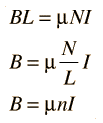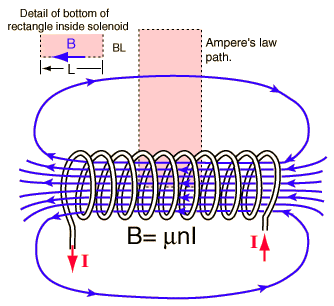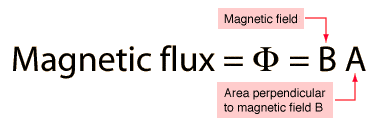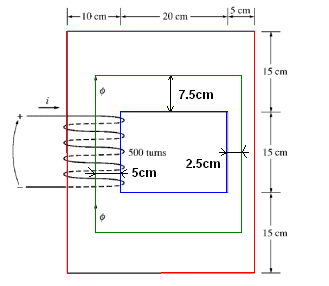# Find the Current and Flux Density in a Feromagnetic Core

## Homework Statement

A ferromagnetic core is shown the figure below. The depth of the core is 5 cm. The other
dimensions of the core are as shown in the figure. Find the value of the current that will produce a flux of 0.003 Wb. With this current, what is the flux density at the top of the core? What is the flux density at the right side of the core? Assume that the relative permeability of the core is 1000.

R=l/μA

## The Attempt at a Solution

So I know to use this equation R=l/μA and I get what μ is and based on a similar problem in my book I also know what to do after I get Req. However what I am stuck on is how to get the mean path length l and the cross sectional areas A. I also know from the problem in the book I am supposed to seperate it into different regions but how exactly do I do this?

#### Attachments

•Capture.PNG
4.3 KB · Views: 1,392

## Homework Statement

A ferromagnetic core is shown the figure below. The depth of the core is 5 cm. The other
dimensions of the core are as shown in the figure. Find the value of the current that will produce a flux of 0.003 Wb. With this current, what is the flux density at the top of the core? What is the flux density at the right side of the core? Assume that the relative permeability of the core is 1000.

R=l/μA

## The Attempt at a Solution

So I know to use this equation R=l/μA and I get what μ is and based on a similar problem in my book I also know what to do after I get Req. However what I am stuck on is how to get the mean path length l and the cross sectional areas A. I also know from the problem in the book I am supposed to seperate it into different regions but how exactly do I do this?

This can help youhttp://hyperphysics.phy-astr.gsu.edu/hbase/magnetic/solenoid.html

andhttp://hyperphysics.phy-astr.gsu.edu/hbase/magnetic/fluxmg.html#c1

And be careful with units

Last edited by a moderator:
You can find the mean length of the flux path very easy.The red line represents the longest path which a flux line could take to go round the core. The blue line is the shortest path. Shown in green is the mean length of the flux path.
le = (l_red + l_blue)/2

l_red = 2*(10cm + 20cm + 5cm) +2*(15cm + 15cm + 15cm) = 160cm

l_blue = 2*20cm + 2*15cm = 70cm

So

le = (160cm + 70cm)/2 = 115cm

#### Attachments

Thanks for the help I will look at this later when I have more time and let you know if I have problems.

rude man
Homework Helper
Gold Member
You don't want to compute mean path lengths. You want to compute the reluctance around the core.

You had the right formula for reluctance: R = l/μA.

So compute the sum of this formula for the four sections of the core: R = ∑ Ri = (1/μ)∑ li/Ai, i = 1 to 4.

Then apply mmf = Rø. ø = BA = flux and is constant around the core. mmf is magnetomotive force = NI. I is current.

ok so I get the length stuff now but I still dont understand how the areas are being produced I know for a rectangular space its length * width but whenever I do it the answer is wrong. I keep thinking the area for the top is 7.5*27.5=206.25 this is obviously wrong what am I doing. Should I be taking the coil dimensions?

I have l1= 55 cm and l2=l3=30 cm

rude man
Homework Helper
Gold Member
ok so I get the length stuff now but I still dont understand how the areas are being produced I know for a rectangular space its length * width but whenever I do it the answer is wrong. I keep thinking the area for the top is 7.5*27.5=206.25 this is obviously wrong what am I doing. Should I be taking the coil dimensions?

The area for the top is 15cm x 5cm = 75 cm2. It's the cross-sectional area you're looking for.

Where are you taking the cross section from that looks like the right what am I not seeing?

rude man
Homework Helper
Gold Member
Where are you taking the cross section from that looks like the right what am I not seeing?

The cross-section on the right leg is 5 x 5 = 25 cm^2.
The flux does not flow into or out of the page. It flows along the perimeter with depth = 5 cm everywhere.

BTW you CAN do the problem by using the mean free path and doing some fancy area integration along the bends. That might even be more accurate than what I suggested. With either method the trick is to approximate the path area along the bends.

Usually in a magnetic circuit like this there is an air gap which allows approximating R by d/μ0A where d = gap length and A = gap area. Reason is that μ is much greater in the paramagnetic (iron, steel etc.) core than in the air gap. So the length of the path in tghe core is immaterial. Unfortunately in this problem there is no such gap.

Last edited:
ok so the left would be 10*5=50 because 10 cm is the diameter and 5 cm is the depth.

If I read what you put right it is 5*5 because it has a 5cm radius and 5 cm depth.

rude man
Homework Helper
Gold Member
"Radius" and "diameter" refer to circular cross-sections. These cross-sections are rectangular.

The left cross-section is 10 x 5. The top & bottom are 15 x 5. The right is 5 x 5.

oh herp derp I got it now alright so back to the equation R1=l1/μA1=.55/((4pix10^-7)(1000)(.0075))=58.36, R2=l2/μA2=.30/((4pix10^-7)(1000)(.0025))=95.49, and R3=l3/μA3=.30/((4pix10^-7)(1000)(.0050))=47.74 the Req=R1+R2+R3= 58.36+95.49+47.74=201.59 then P=1/Req=1/201.59=.004960 then ø=FP so F=ø/P=.003/.004960=.604. F=Ni so .604=500i which gives I=1.2A then B=ø/A3=.003/.0075=0.4 T and B=ø/A1=.003/.0025=1.2 T

Yah so its just basically rectangles 5 cm is on the right times its depth so that gives 5x5 on the left you have 10 and depth of 5 so 10*5 and top and bottom is 15*5 because 15 is at top and bottom

rude man
Homework Helper
Gold Member
Yah so its just basically rectangles 5 cm is on the right times its depth so that gives 5x5 on the left you have 10 and depth of 5 so 10*5 and top and bottom is 15*5 because 15 is at top and bottom

Yes.

Can't read your previous post.

When you compute the path lengths don't count the corners twice!
Total length is 25 + 25 + 15 +15.

I got the same question.but how to calculate the length at the top??
20cm+5cm+2.5cm=27.5cm..

I have l1= 55 cm and l2=l3=30 cm
How l1=55

How l1=55

HOW DID U GET THE AREAS FOR EACH AREA THAT U USED TO FIND THEIR RELUCTANCES